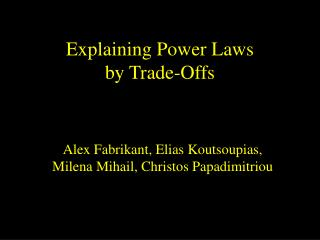Download Presentation# Explaining Power Laws by Trade-Offs

Download Presentation## Explaining Power Laws by Trade-Offs

- - - - - - - - - - - - - - - - - - - - - - - - - - - E N D - - - - - - - - - - - - - - - - - - - - - - - - - - -
##### Presentation Transcript

1. Explaining Power Laws by Trade-Offs Alex Fabrikant, Elias Koutsoupias, Milena Mihail, Christos Papadimitriou

2. Powerlaws in the Internet • [Faloutsos3 1999]: the degrees of the Internet topology are power law distributed • Both autonomous systems graph and router graph • Hop distances: ditto • Eigenvalues: ditto (!??!) • Model? DIMACS, Feb 13, 2002

3. The world according to Zipf • Power laws, Zipf’s law, heavy tails,… • “the signature of human activity” • i-th largest is ~ i-a (cities, words: a = 1) • Equivalently: prob[X greater than x] ~ x -b • (compare with law of large numbers) DIMACS, Feb 13, 2002

4. Models predicting power laws • Size-independent growth (“the rich get richer”) • Preferential attachment • Brownian motion in log • Exponential arrival + exponential growth • Copying (web graph) • Carlson and Doyle 1999: Highly optimized tolerance (HOT) DIMACS, Feb 13, 2002

5. Our model: minj < i [  dij + hopj] DIMACS, Feb 13, 2002

6. hopj • Average hop distance from other nodes • Maximum hop distance from other nodes • Distance from center (first node) NB: Resulting graph is a tree DIMACS, Feb 13, 2002

7. Theorem: • if  < const, then graph is a star degree = n -1 • if  > n, then there is exponential concentration of degrees prob(degree > x) < exp(-ax) • otherwise, if const <  < n, heavy tail prob(degree > x) > x -a DIMACS, Feb 13, 2002

8. Also: why are files on the Internet power-law distributed? • Suppose each data item i has “popularity” ai • Partition data items in files to minimize total cost • Cost of each file: total popularity · size + overhead C • Notice trade-off! • From [CD99] DIMACS, Feb 13, 2002

9. Files (continued) • Suppose further that popularities of items are iid from distribution f • Result: File sizes are power law distributed “for any reasonable” distribution f (exponential, Gaussian, uniform, power law, etc.) • ([CD99] observe it for a few distributions) DIMACS, Feb 13, 2002

10. Heuristically optimized tradeoffs • Power law distributions seem to also come from tradeoffs between objectives (asignature of human activity?) • Generalizes [CD99] (the other objective need not be reliability) • cf [Mandelbrot 1954]: Power Laws in language are due to a tradeoff between information and communication costs DIMACS, Feb 13, 2002

11. PS: Eigenvalues of the Internet may be a corollary of the degrees phenomenon: Theorem: If a graph has largest degrees d1, d2,…, dk and o(dk ) more edges, then with high probability its largest eigenvalues are within (1+ o(1)) of d1, d2,…, dk (NB: The eigenvalue exponent observed in Faloutsos3 is about ½ of the degree exponent!) DIMACS, Feb 13, 2002